# Images of the Experiment

## Setup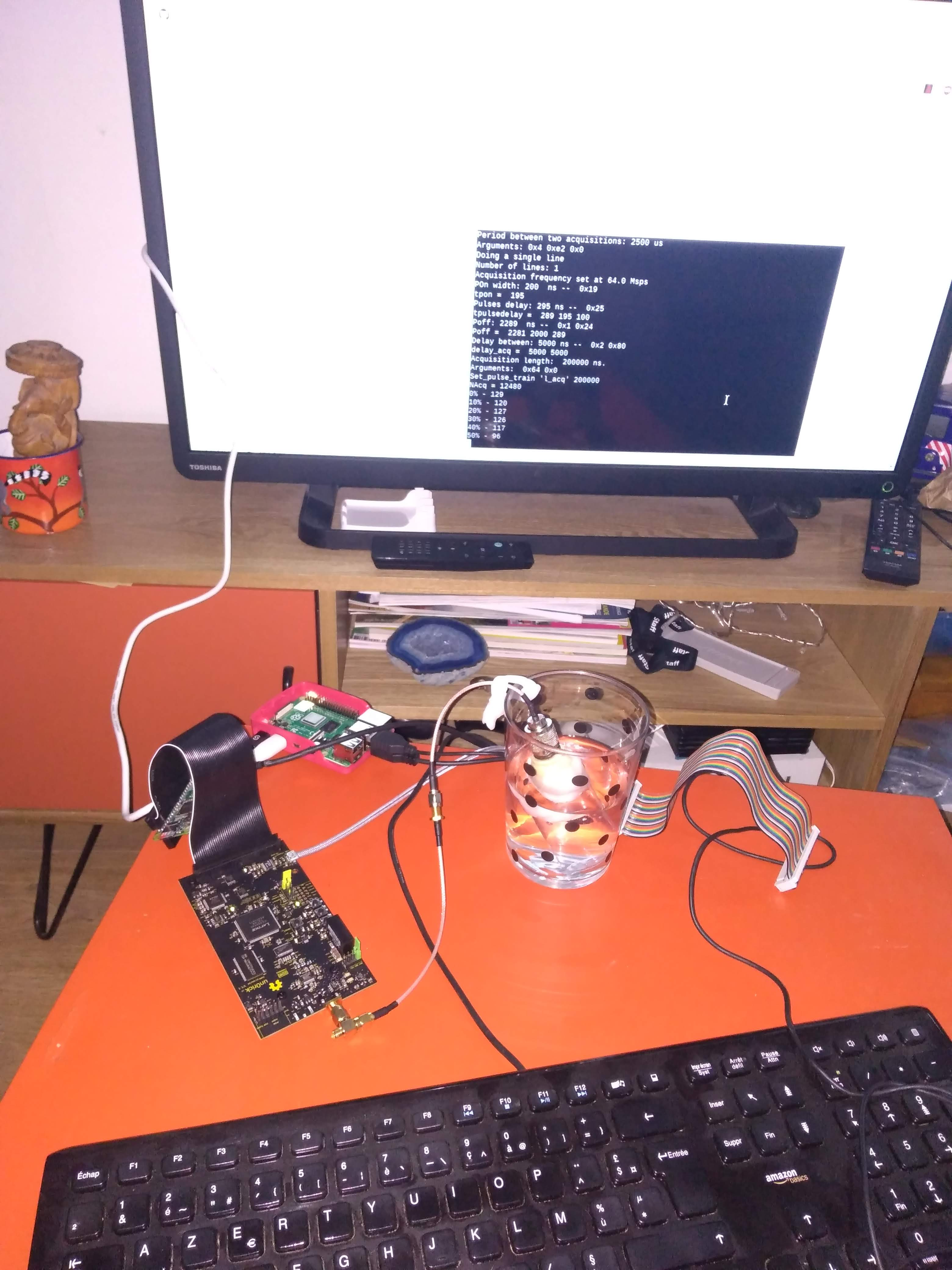Test of the new batches

## Others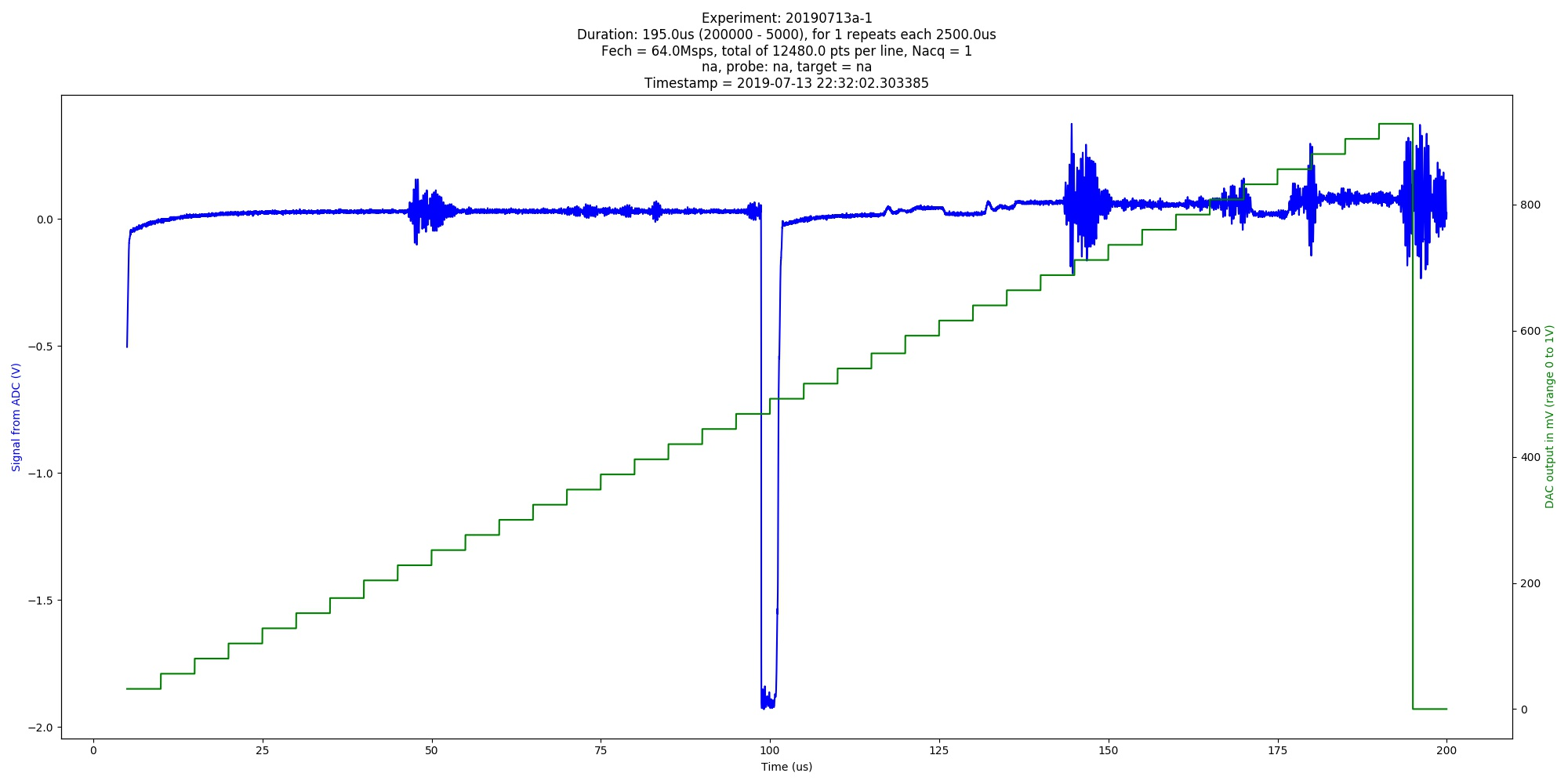Automated image of 20190713a experiment. na (category: graph).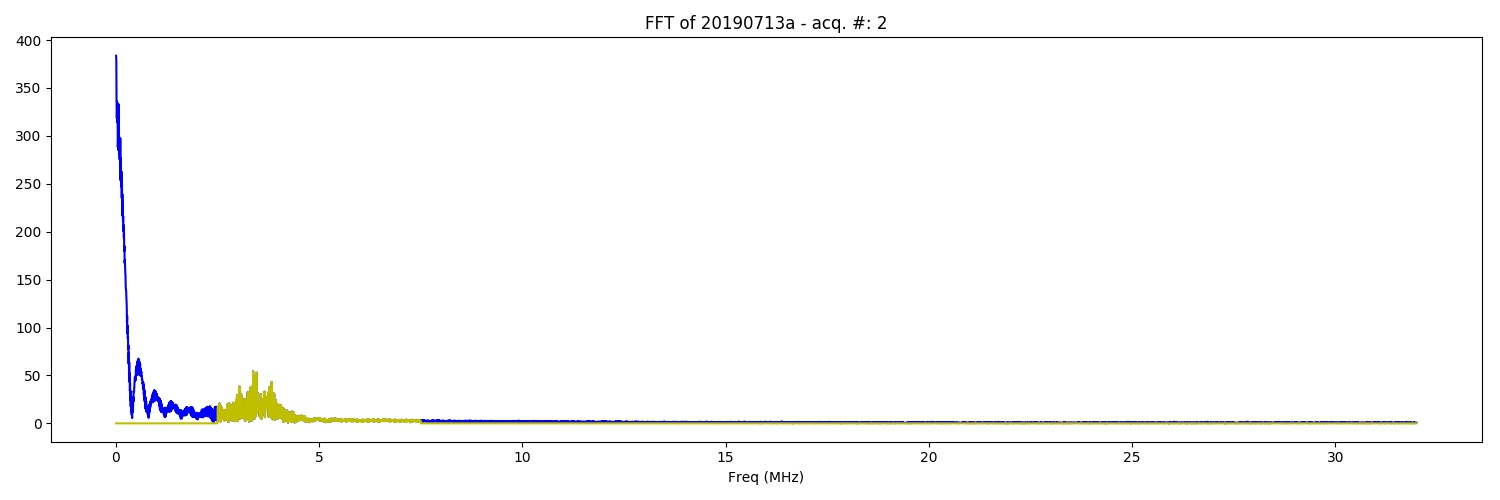FFT of the of 20190713a experiment. na (category: FFT).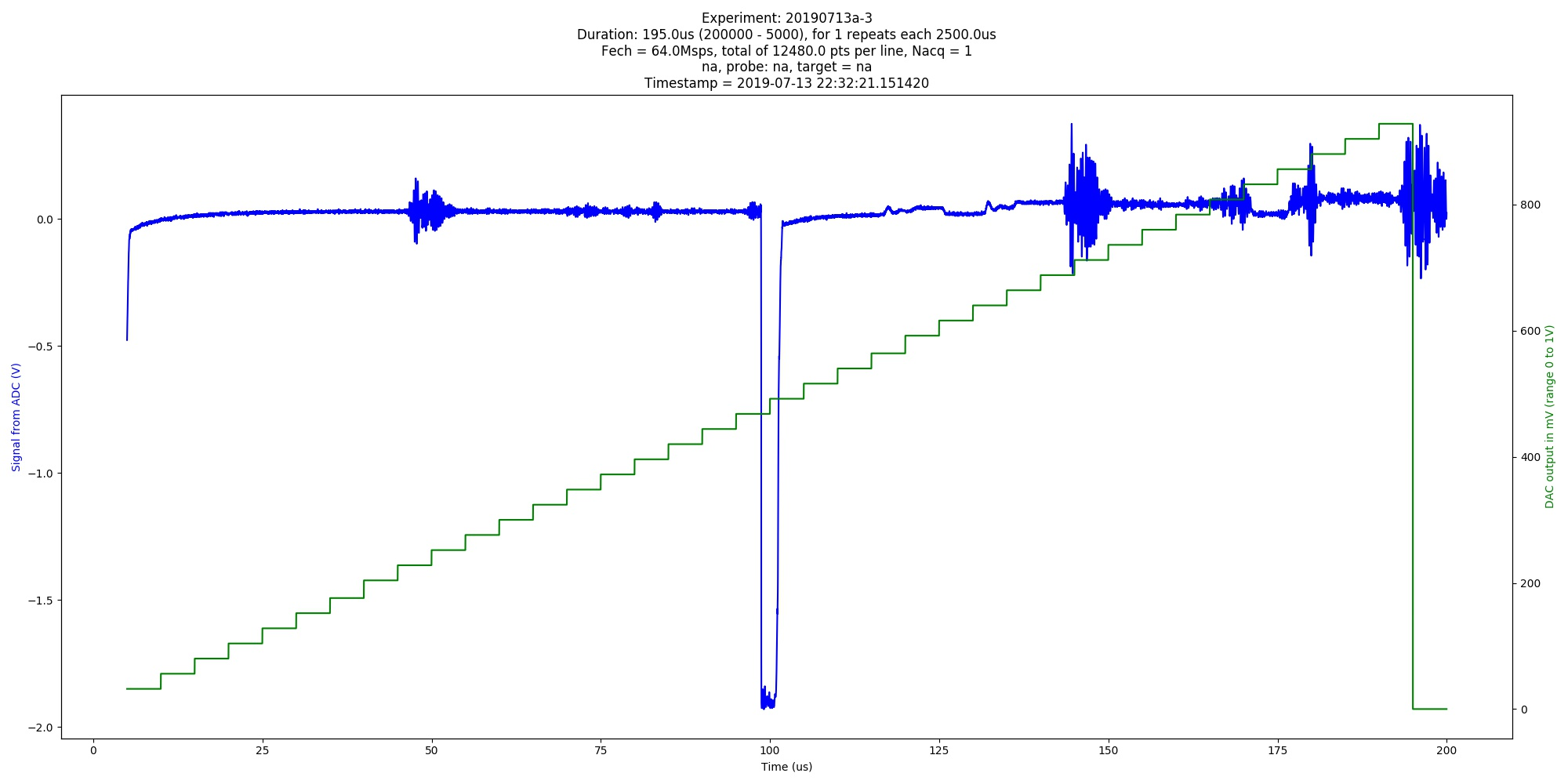Automated image of 20190713a experiment. na (category: graph).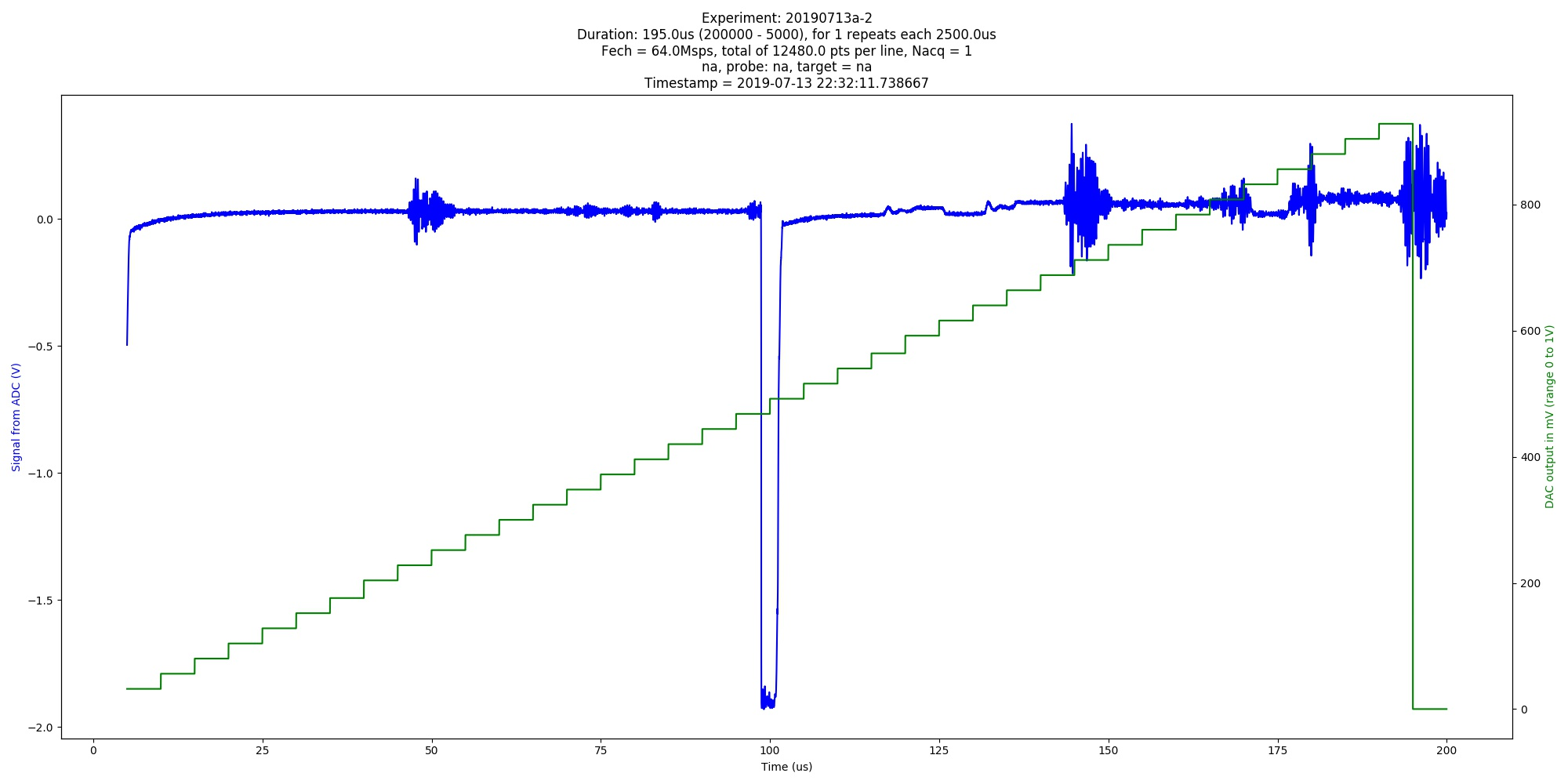Automated image of 20190713a experiment. na (category: graph).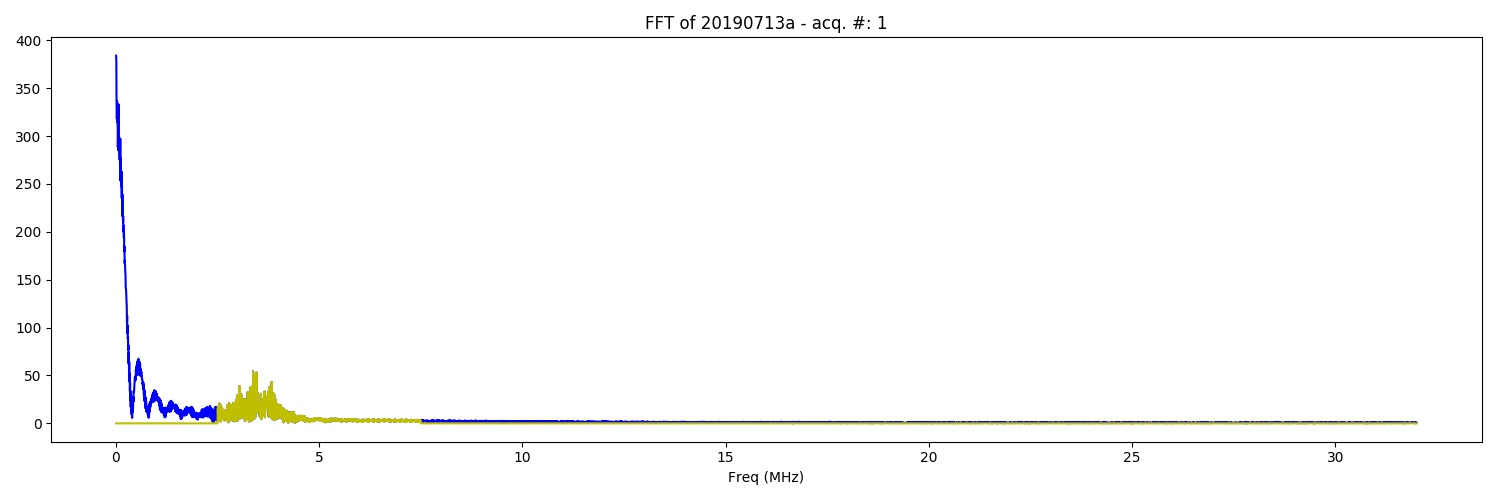FFT of the of 20190713a experiment. na (category: FFT).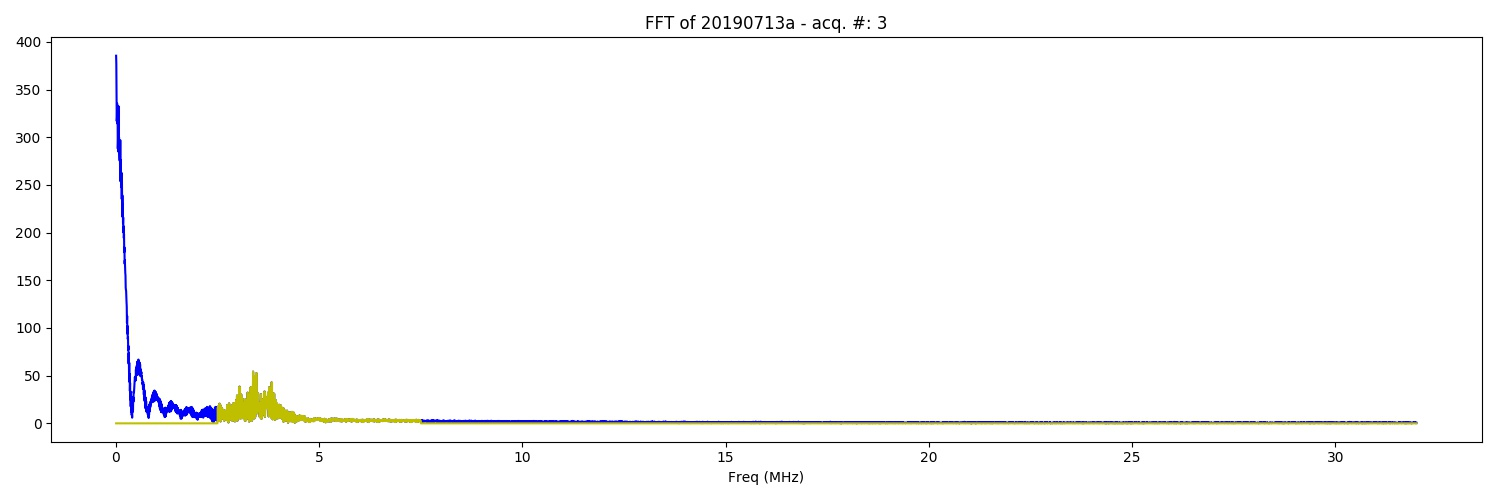FFT of the of 20190713a experiment. na (category: FFT).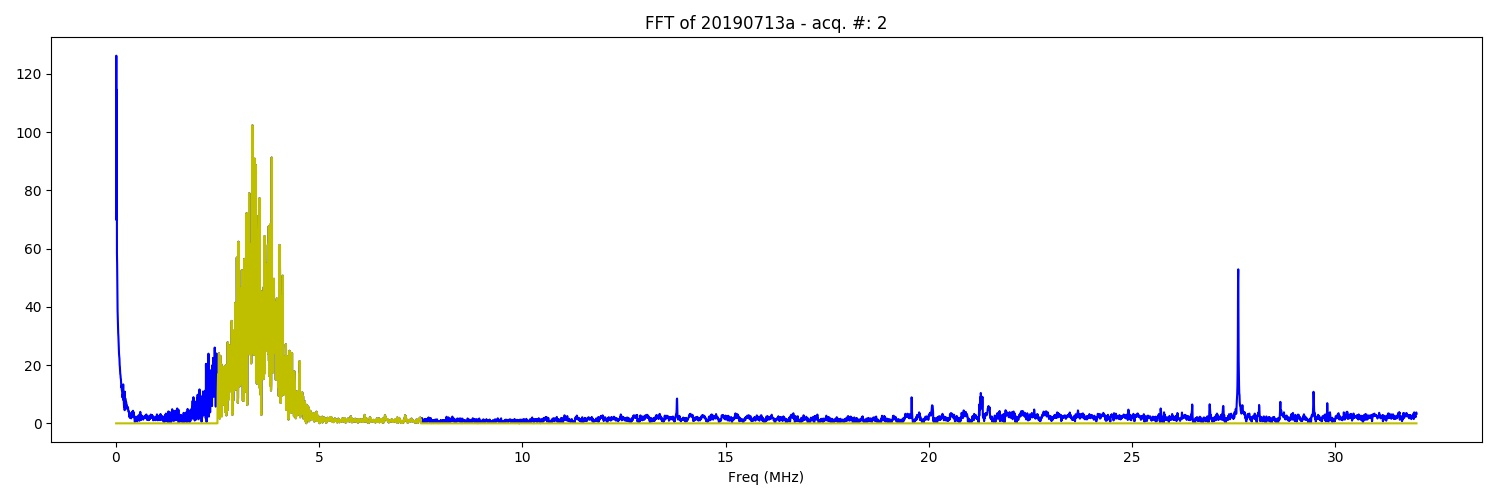FFT of the of 20190713a experiment. na (category: FFT).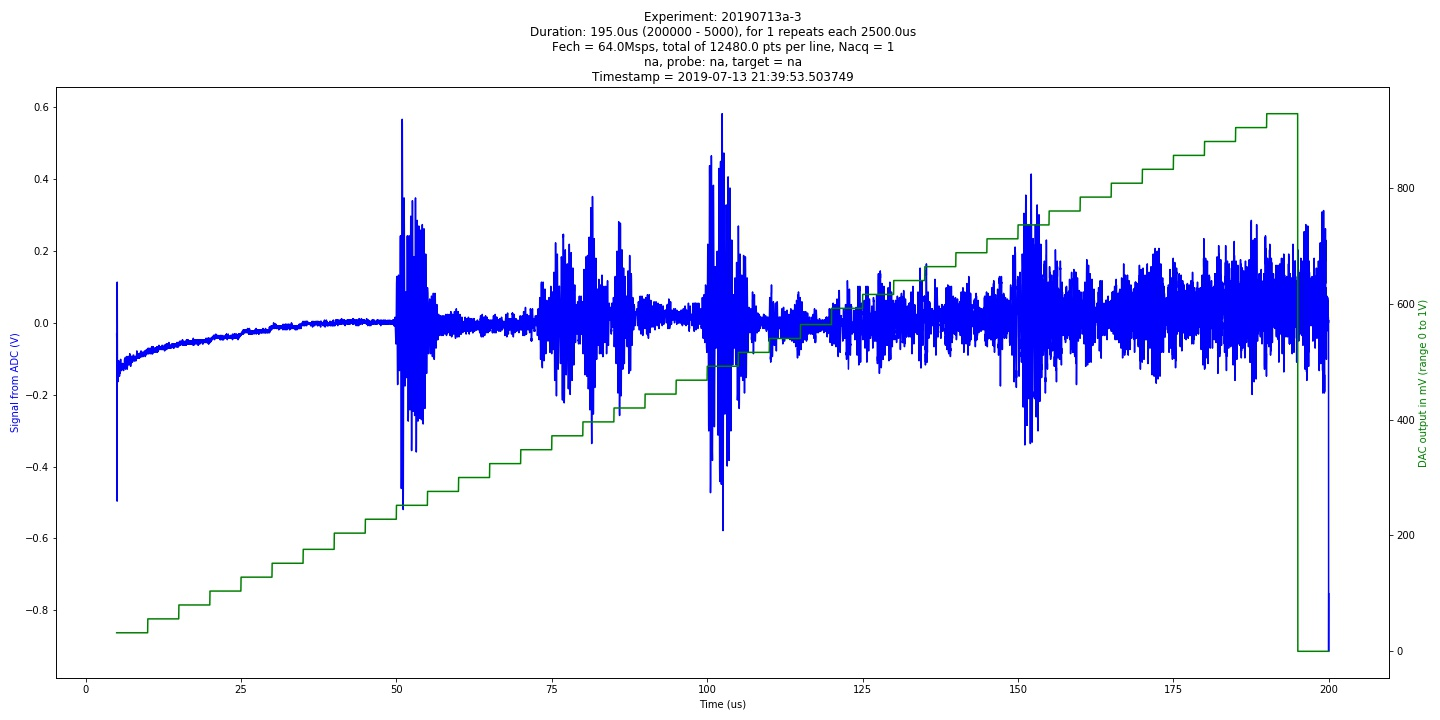Automated image of 20190713a experiment. na (category: graph).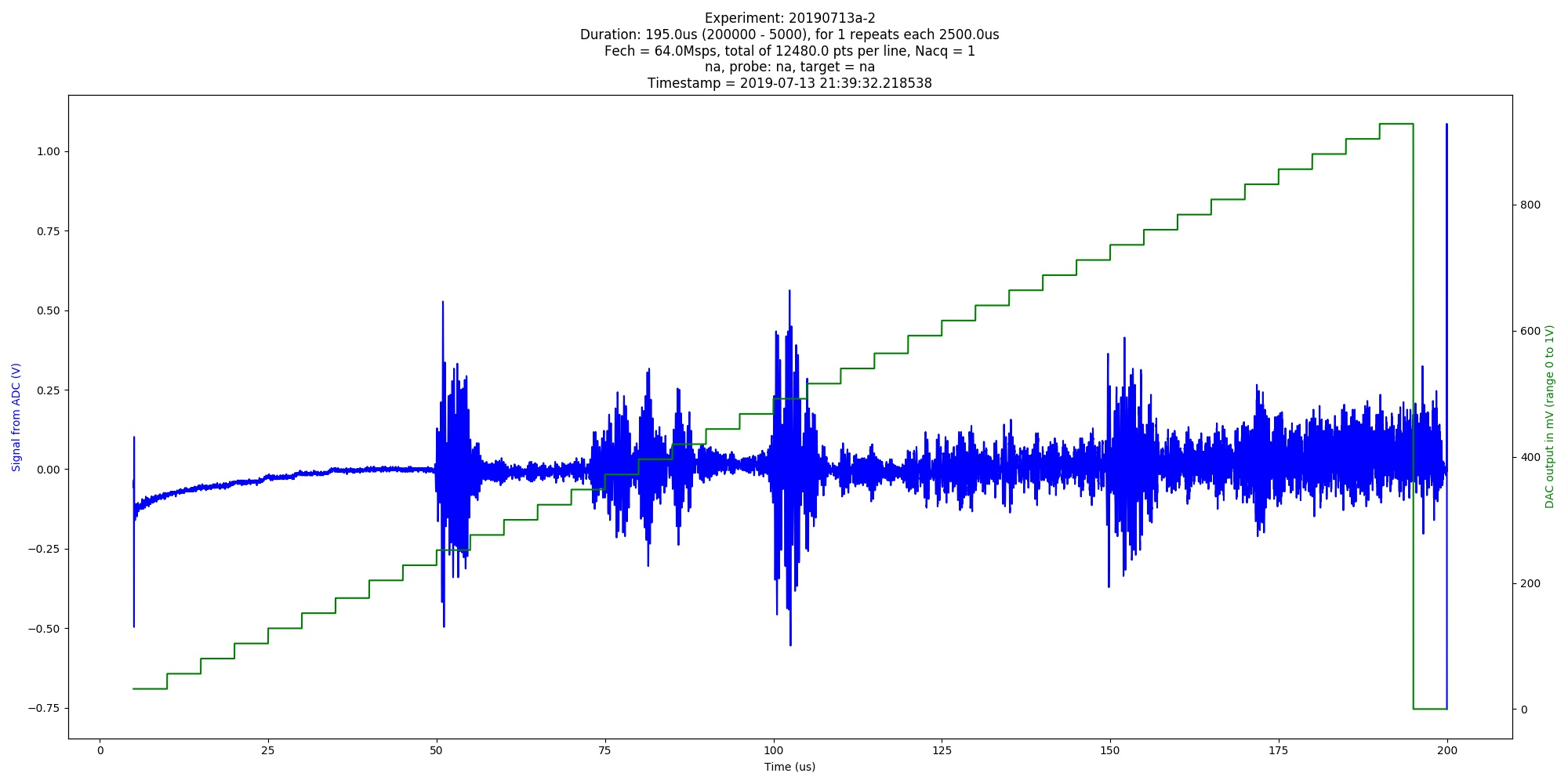Automated image of 20190713a experiment. na (category: graph).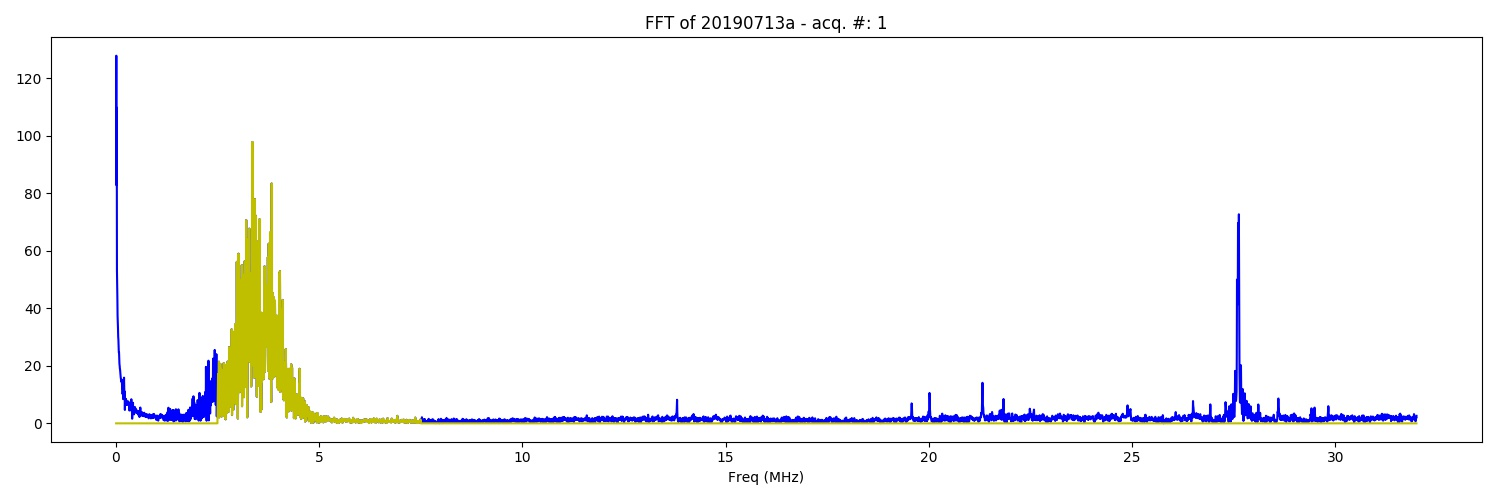FFT of the of 20190713a experiment. na (category: FFT).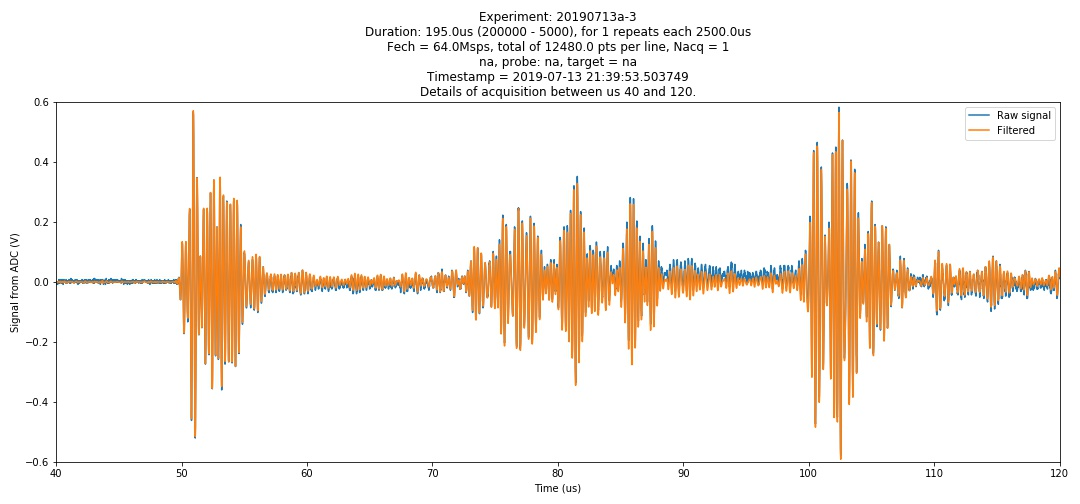Experiment: 20190713a-3 Duration: 195.0us (200000 - 5000), for 1 repeats each 2500.0us Fech = 64.0Msps, total of 12480.0 pts per line, Nacq = 1 na, probe: na, target = na Timestamp = 2019-07-13 21:39:53.503749 Details of acquisition between us 40 and 120. (category: graph).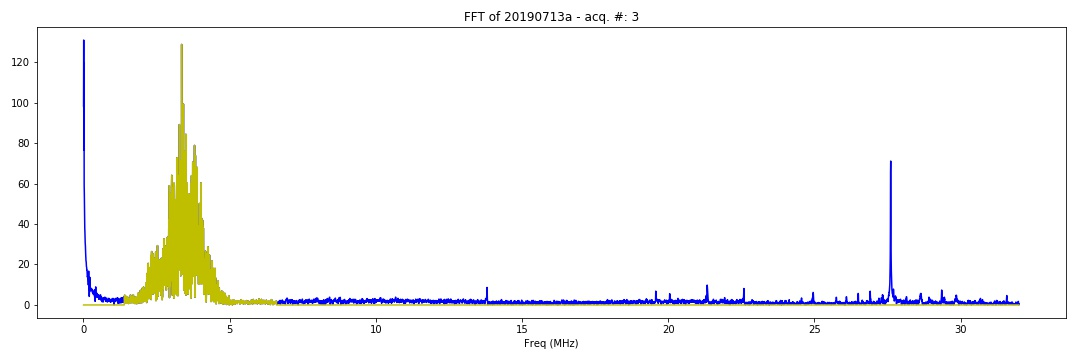FFT of the of 20190713a experiment. na (category: FFT).